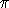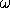## CGAL::Regular_triangulation_2<Traits,Tds>

### Definition

The class Regular_triangulation_2<Traits,Tds> is designed to maintain the regular triangulation of a set of weighted points.

Let  PW = {(pi, wi), i = 1, ..., n } be a set of weighted points where each pi is a point and each wi is a scalar called the weight of point pi. Alternatively, each weighted point (pi, wi) can be regarded as a two dimensional sphere with center pi and radius ri=sqrt(wi).

The power diagram of the set  PW is a planar partition such that each cell corresponds to sphere (pi, wi) of  PW and is the locus of points p whose power with respect to (pi, wi) is less than its power with respect to any other sphere (pj, wj) in  PW. The dual of this diagram is a triangulation whose domain covers the convex hull of the set  P= { pi, i = 1, ..., n } of center points and whose vertices are a subset of  P. Such a triangulation is called a regular triangulation. The three points pi, pj and pk of  P form a triangle in the regular triangulation of  PW iff there is a point p of the plane whose powers with respect to (pi, wi), (pj, wj) and (pk, wk) are equal and less than the power of p with respect to any other sphere in  PW.

Let us defined the power product of two weighted points (pi, wi) and (pj, wj) as:(pi, wi,pj, wj) = pipj 2 - wi - wj .(pi, wi,pj, 0) is simply the power of point pj with respect to the sphere (pi, wi), and two weighted points are said to be orthogonal if their power product is null. The power circle of three weighted points (pi, wi), (pj, wj) and (pk, wk) is defined as the unique circle (,) orthogonal to (pi, wi), (pj, wj) and (pk, wk).

The regular triangulation of the sets  PW satisfies the following regular property (which just reduces to the Delaunay property when all the weights are null): a triangle pipjpk of the regular triangulation of  PW is such that the power product of any weighted point (pl, wl) of  PW with the power circle of (pi, wi), (pj, wj) is (pk, wk) is positive or null. We call power test of the weighted point (pl, wl) with respect to the face pipjpk, the predicates testing the sign of the power product of (pl, wl) with respect to the power circle of (pi, wi), (pj, wj) is (pk, wk). This power product is given by the following determinant

|
 1 xi yi xi 2 + yi 2 - wi 1 xj yj xj 2 + yj 2 - wj 1 xk yk xk 2 + yk 2 - wk 1 xl yl xl 2 + yl 2 - wl
|

A pair of neighboring faces pipjpk and pipjpl is said to be locally regular (with respect to the weights in  PW) if the power test of (pl,wl) with respect to pipjpk is positive. A classical result of computational geometry establishes that a triangulation of the convex hull of  P such that any pair of neighboring faces is regular with respect to  PW, is a regular triangulation of  PW.

Alternatively, the regular triangulation of the weighted points set  PW can be obtained as the projection on the two dimensional plane of the convex hull of the set of three dimensional points  P'= { (pi,pi 2 - wi ), i = 1, ..., n }.

The vertices of the regular triangulation of a set of weighted points  PW form only a subset of the set of center points of  PW. Therefore the insertion of a weighted point in a regular triangulation does not necessarily imply the creation of a new vertex. If the new inserted point does not appear as a vertex in the regular triangulation, it is said to be hidden.

Hidden points are stored in special vertices called hidden vertices. A hidden point is considered as hidden by the facet of the triangulation where its point component is located : in fact, the hidden point can appear as vertex of the triangulation only if this facet is removed. Each face of a regular triangulation stores the list of hidden vertices whose points are located in the facet. When a facet is removed, points hidden by this facet are reinserted in the triangulation.

#include <CGAL/Regular_triangulation_2.h>

### Parameters

The geometric traits parameter Traits has to be instantiated with a model of the concept RegularTriangulationTraits_2. The concept RegularTriangulationTraits_2 refines the concept TriangulationTraits_2 by adding the type Weighted_point_2 to describe weighted points and the type Power_test_2 to perform power tests on weighted points.

The Tds parameter has to be instantiated by a model of TriangulationDataStructure_2. The face base of a regular triangulation has to be a model of the concept RegularTriangulationFaceBase_2. while the vertex base class has to be a model of RegularTriangulationVertexBase_2. CGAL provides a default instantiation for the Tds parameter by the class CGAL::Triangulation_data_structure_2 < CGAL::Reugular_triangulation_vertex_base_2<Traits>, CGAL::Regular_Triangulation_face_base_2<Traits> >.

### Inherits From

Triangulation_2<Traits,Tds>

### Types

 typedef Traits::Distance Distance; typedef Traits::Line Line; typedef Traits::Ray Ray; typedef Traits::Bare_point Bare_point; typedef Traits::Weighted_point Weighted_point;

 Regular_triangulation_2::All_vertices_iterator An iterator that allows to enumerate the vertices that are not hidden. Regular_triangulation_2::Finite_vertices_iterator An iterator that allows to enumerate the finite vertices that are not hidden. Regular_triangulation_2::Hidden_vertices_iterator; An iterator that allows to enumerate the hidden vertices.

### Creation

 Regular_triangulation_2 rt ( Traits gt = Traits()); Introduces an empty regular triangulation rt. Regular_triangulation_2 rt ( Regular_triangulation_2 rt); Copy constructor.

### Insertion and Removal

Vertex_handle
 rt.insert ( Weighted_point p, Face_handle f=Face_handle())
inserts weighted point p in the regular triangulation. If the point p does not appear as a vertex of the triangulation, the returned vertex is a hidden vertex. If given the parameter f is used as an hint for the place to start the location process of point p.

Vertex_handle
 rt.insert ( Weighted_point p, Locate_type lt, Face_handle loc, int li)
insert a weighted point p whose bare-point is assumed to be located in lt,loc,li.

Vertex_handle rt.push_back ( Point p)
Equivalent to insert(p).

template < class InputIterator >
int rt.insert ( InputIterator first, InputIterator last)
inserts the weighted points in the range [.first, last.). Returns the number of created vertices.
Precondition: The value_type of first and last is Weighted_point.

void rt.remove ( Vertex_handle v)
removes the vertex from the triangulation.

### Queries

template <class OutputItFaces, class OutputItBoundaryEdges, class OutputItHiddenVertices>
CGAL::Triple<OutputItFaces,OutputItBoundaryEdges,OutputItHiddenVertices>
 rt.get_conflicts_and_boundary_and_hidden_vertices ( Weighted_point p, OutputItFaces fit, OutputItBoundaryEdges eit, OutputItHiddenVertices vit, Face_handle start)
OutputItFaces is an output iterator with Face_handle as value type. OutputItBoundaryEdges stands for an output iterator with Edge as value type. OutputItHiddenVertices is an output iterator with Vertex_handle as value type. This member function outputs in the container pointed to by fit the faces which are in conflict with point p i. e. the faces whose power circles have negative power wrt. p. It outputs in the container pointed to by eit the boundary of the zone in conflict with p. It inserts the vertices that would be hidden by p into the container pointed to by vit. The boundary edges of the conflict zone are ouput in counter-clockwise order and each edge is described through its incident face which is not in conflict with p. The function returns in a CGAL::Triple the resulting output iterators.

template <class OutputItFaces, class OutputItBoundaryEdges>
std::pair<OutputItFaces,OutputItBoundaryEdges>
 rt.get_conflicts_and_boundary ( Weighted_point p, OutputItFaces fit, OutputItBoundaryEdges eit, Face_handle start)
same as above except that only the faces in conflict with p and the boundary edges of the conflict zone are output via the corresponding output iterators. The function returns in a std::pair the resulting output iterators.

template <class OutputItFaces, class OutputItHiddenVertices>
std::pair<OutputItFaces,OutputItHiddenVertices>
 rt.get_conflicts_and_hidden_vertices ( Weighted_point p, OutputItFaces fit, OutputItHiddenVertices vit, Face_handle start)
same as above except that only the faces in conflict with p and the vertices that would be hidden by p are output via the corresponding output iterators. The function returns in a std::pair the resulting output iterators.

template <class OutputItBoundaryEdges, class OutputItHiddenVertices>
std::pair<OutputItBoundaryEdges,OutputItHiddenVertices>
 rt.get_boundary_of_conflicts_and_hidden_vertices ( Weighted_point p, OutputItBoundaryEdges eit, OutputItHiddenVertices vit, Face_handle start)
same as above except that only the the vertices that would be hidden by p and the boundary of the zone in conflict with p are output via the corresponding output iterators. The boundary edges of the conflict zone are ouput in counterclockwise order and each edge is described through the incident face which is not in conflict with p. The function returns in a std::pair the resulting output iterators.

template <class OutputItFaces>
OutputItFaces
 rt.get_conflicts ( Point p, OutputItFaces fit, Face_handle start)
same as above except that only the faces in conflict with p are output. The function returns the resulting output iterator.

template <class OutputItBoundaryEdges>
OutputItBoundaryEdges
 rt.get_boundary_of_conflicts ( Point p, OutputItBoundaryEdges eit, Face_handle start)
same as above except that only the boundary edges of the conflict zone are ouput in counterclockwise order where each edge is described through the incident face which is not in conflict with p. The function returns the resulting output iterator.

template <class OutputItHiddenVertices>
OutputItHiddenVertices
 rt.get_hidden_vertices ( Point p, OutputItHiddenVertices vit, Face_handle start)
same as above except that only the vertices that would be hidden by p are output. The function returns the resulting output iterator.

### Access functions

 int rt.number_of_vertices () returns the number of finite vertices that are not hidden. int rt.number_of_hidden_vertices () returns the number of hidden vertices. Hidden_vertices_iterator rt.hidden_vertices_begin () starts at an arbitrary hidden vertex. Hidden_vertices_iterator rt.hidden_vertices_end () past the end iterator for the sequence of hidden vertices. Finite_vertices_iterator rt.finite_vertices_begin () starts at an arbitrary unhidden finite vertex Finite_vertices_iterator rt.finite_vertices_end () Past-the-end iterator All_vertices_iterator rt.all_vertices_end () starts at an arbitrary unhidden vertex. All_vertices_iterator rt.all_vertices_begin () past the end iterator.

### Dual power diagram

The following member functions provide the elements of the dual power diagram.

 Point rt.weighted_circumcenter ( Face_handle f) returns the center of the circle orthogonal to the three weighted points corresponding to the vertices of face f. Precondition: f is not infinite Point rt.dual ( Face_handle f) same as weighted_circumcenter Object rt.dual ( Edge e) If both incident faces are finite, returns a segment whose endpoints are the duals of each incident face. If only one incident face is finite, returns a ray whose endpoint is the dual of the finite incident face and supported by the line which is the bisector of the edge's endpoints. If both incident faces are infinite, returns the line which is the bisector of the edge's endpoints otherwise. Object rt.dual ( Edge_circulator ec) Idem Object rt.dual ( Edge_iterator ei) Idem template < class Stream> Stream& rt.draw_dual ( Stream & ps) output the dual power diagram to stream ps.

### Predicates

 Oriented_side rt.power_test ( Face_handle f, Weighted_point p) Returns the power test of p with respect to the power circle associated with fadvanced### Miscellaneous

 bool rt.is_valid ( bool verbose = false, int level = 0) Tests the validity of the triangulation as a Triangulation_2 and additionally test the regularity of the triangulation. This method is useful to debug regular triangulation algorithms implemented by the user.advanced